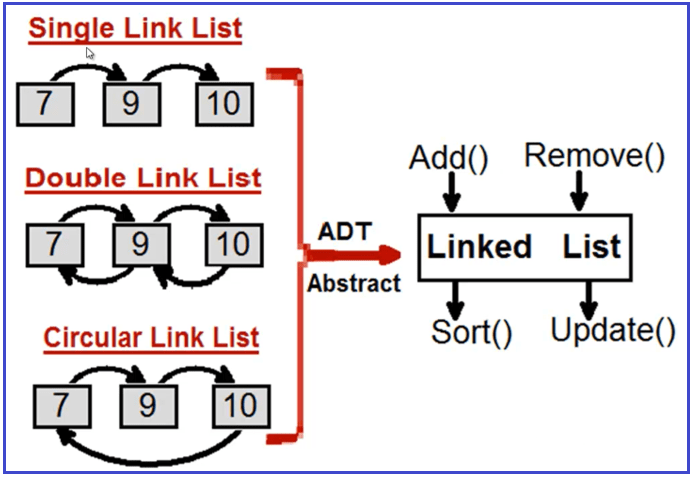In this article, we will discuss the Abstract Data Type that is ADT. Please read our previous article where we discussed Stack vs Heap Memory in detail. Let us first understand what is data type and then we will learn abstract data type.

##### What does it mean by data type?

A data type is defined as

1. Representation of data and
2. Operations on data,

So, a data type is defined in two terms i.e. first thing is how the data is represented or how you are storing the data. The second thing is what are the operations that we allow on the data.

So, if we take an example of integer data type in C or C++ languages, then if we assume that integer data type takes 2 bytes in C or C++, once we declare any variable of type integer in C or C++, then we get the memory of 2-bytes that is 16-bits.

An integer type of data is stored in 2-bytes together as a single value or single data. In which 1-bit is reserved as sign bit that allow both positive, as well as negative number and rest of the 15-bits, are allowed for storing data that is any number. This is how an integer is represented inside the memory in 2-bytes. For better understanding, please have a look at the following image.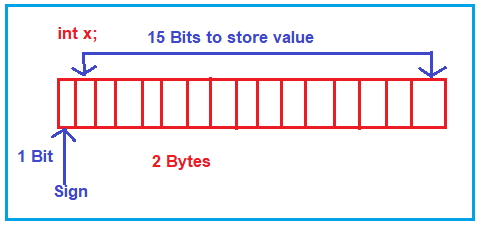So, this is the first thing we have discussed i.e. the representation of data. Now let us understand what all operations can be performed on the integer data type.

##### What are the operations allowed on integer type data in C, C++ language?

Arithmetic operations are allowed on integer type data, that is +, -, *, / and %. Apart from these, relational operation i.e. >, <, >=, <= as well as increment and decrement operators that is ‘++’ or ‘- -‘ are allowed. These are some of the sets of operations that are allowed on the integer data type.

So, if we talk about data type, any data type in any language then that data type will have its representations and the set of operations that can be performed on the data. When we are learning any programming language, when we learn any data type, we learn mostly about its operations and sometimes we go into detail and also understand its representation.

##### What is Abstract?

The abstract means hiding internal details. For better understanding please have a look at the following image which shows the representation and operations that can be performed on integer data. For performing the operations (+, -, *, /, %, ++, –) do we really need to know, how they are performed in the binary from inside the main memory? The answer is No. We are only concerned about declaring a variable and using it by performing those operations. So, we need not know the internal details of how these operations are performed. These things are hidden from us. So, we can call them Abstract i.e. without knowing internal details we can use it. Internal details are Abstract for us.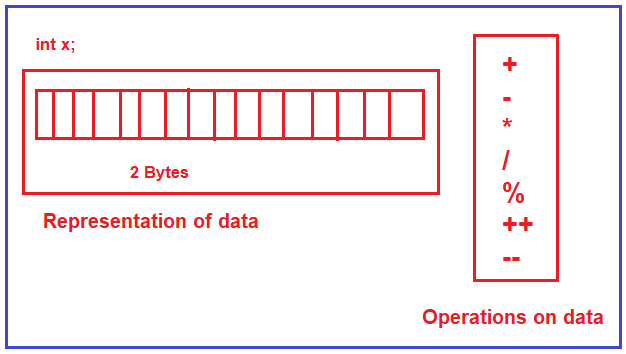The above is the example of a primitive data type I have taken and explain to you the meaning of data type and the meaning of abstract. This is not an abstract data type, that is a primitive data type. But first I try to explain to you the meaning of data type and abstract and I hope you understand this. Let us proceed further and try to understand the Abstract Data Type.

##### Why is Abstract Data Type introduced and what is the meaning of Abstract Data Type?

Abstract Data Type concept is related to Object-Oriented Programming languages. When the Object programming languages being started used in software Development then using the classes, we can define our own data types. That is Abstract, that is without knowing internal details we can use them. Let us take an example of a list and define it as Abstract Data Type i.e. list of elements or collection of elements.

List-> 8, 3, 9, 4, 6, 10, 12

So, here we have taken the list of elements. Now, I can give the indices either starting from 0 or 1 that depends on my requirement. Here, I will start indices from 0 onwards.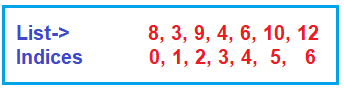Now, I want this list to be used in my program. Now the question is how I can represent a list? What are the things that I have to store for representing the list? We need the following things.

1. Space for storing the elements.
2. The second thing is, we need to have some capacity of a list,
3. The third is, inside that capacity how many elements already we have in the list, that is length of list or size of a list

For representing a list, we need three things. Space for storing the elements and its capacity that is the maximum capacity and its size which is the number of elements it is having. So, for the representation of this, we have two options. We can use either array or linked list in a program. So, it can be done using any of these methods (array and linked list). Then let us look at operations on a list.

##### What are the operations that we perform on a list?

The operations that can be performed on a list are as follows. Here we are adding some of the operations.

2. Remove(x): Remove an element from the list
3. Search(key): To search a particular element from the list

So, when we have two things, data representations and operations on the data together, then it becomes a data type. Now we can put the above data representation and operations together and we can define a class in C++ or in any other Object-Oriented Programming language. For better understanding, please have a look at the following image.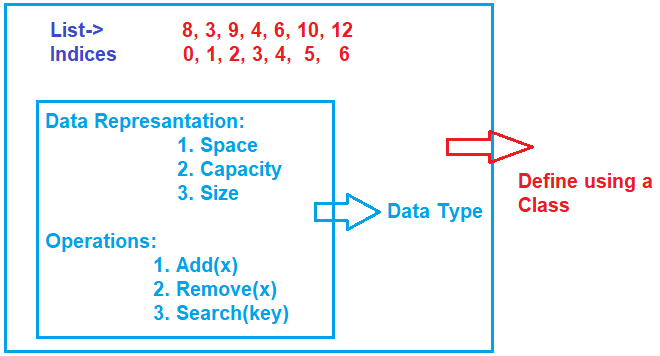##### How we will be storing this list of elements?

Either Array or Linked List. Whatever is used this is going to work perfectly and we’ll be performing the set operations.

How the presentation is done, I need not bother about it. When the class is written, I can just create the object of the class and use it. How internally things are working, I need not be a worry. That’s, what is Abstract.

So, the concept of ADT defines the data and the operations on the data together and let it be used as a data type by hiding all the internal details. This concept of ADT is very common in C++. So, we can say that when we write any class in C++ which has the data presentation and operations together it defines an ADT.

In this course, we are going to learn about various data structures like an array, linked list, stack, queues, graphs, trees, Hash Tables, and all these things we will try to represent them as ADT. So, I will be seeing the code for C language as well as for C++ and then we will see how ADT is implemented. We will be defining all these data structures as ADT (Abstract Data Type) through C++.

##### Operations on a list:

We will look at the operations on a list one by one.

This is used for adding an element to the end of a list. For example, if we want to add 15, then it should be added to the list at the index position 7. This add can also be called as append an element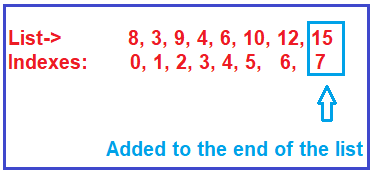###### Add(Index, Element) / Insert(Index, Element)

This is used for adding an element at a given index. For example, if we want to add 20 at index 6. But in index position 6, 12 is there. Then we should shift the elements and make a free space for 20. It means we should move 15 to the next place i.e. index 8 and we should bring 12 to index 7 and then we should insert 20 in index 6. So, if we want to insert any element at a given index then we have to shift the elements. This can also be called as insert at a given index.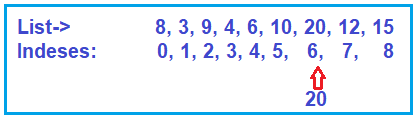##### Remove(index)

This is used for removing an element and the element which you want to remove, you must give that element index number. For example, if we want to remove 20 i.e. index 6, and when we remove 20 that place will be vacant in the list. Then we have to shift the rest of the elements. So, we will be having a total of 8 elements index from 0 to 7, removing one element rest of the elements are still part of the list.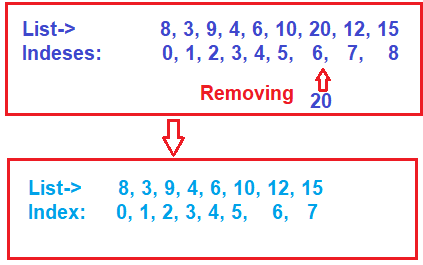###### Set(index, element) / Replace(index, element)

This is used for changing an element at a given index. Suppose you want to change an element at index 3 to 25. The set can also be called replace, which is replacing an element at a given index with a new element.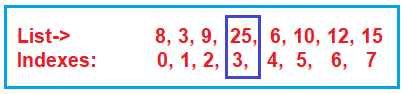###### Get(index)

If you want to know the element at a given index then this method you need to use. For example, if you want to know what is there at index 5. Then it will return the value of 10. So, knowing an element from a given index.

###### Search(key) / Contains(key)

This is used for searching an element with a given key i.e. searching an element in a list. For example, if you want to search for element 9, yes, it is found there at index 2. The result of the search is we get the index. We know element 9, we want to search where it is in the list, it is at index 2. The search is also called as contains. So, we want to know whether the key element is there in the list or not i.e. the list contains that element or not.

###### Sort()

This is used for sorting the list i.e. it is used to arrange all the elements in some.

These are the few operations on a list. There are other operations that we can perform such as reverse the list and when there is more than one list, you can combine them or you can merge them or you can split a list. So, a lot of other operations you can perform on a list. As a part of this ADT article, we have taken an example of a list and we have shown, how it is represented and what are the operations.

##### Understanding Abstract Data Type (ADT):

In today’s world, there are more than 500 to 600 known data structures. Some of them are showing in the below diagram. Nothing about it, you have a situation and you have to go and choose the best data structure suitable for your needs.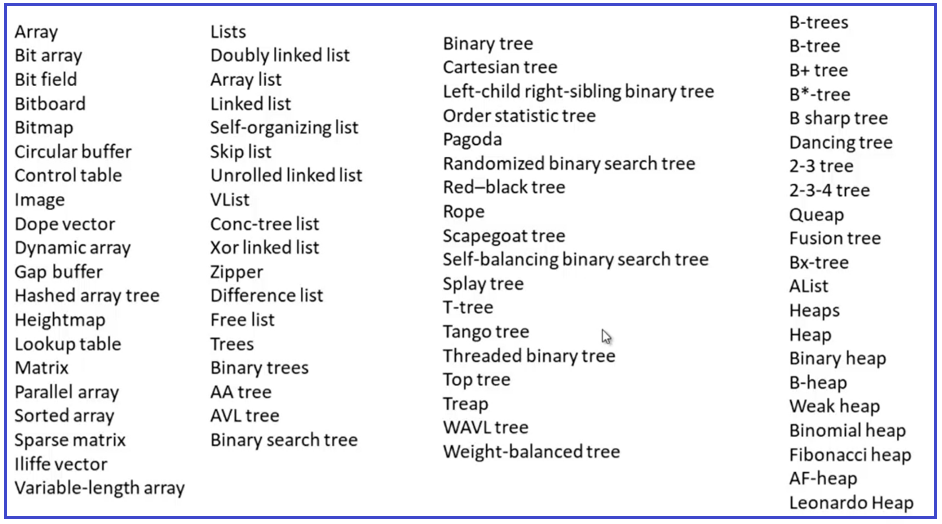And it’s really very difficult to remember each of these data structures. So, if you can go and categories them or you can think in an abstract way that would be great. For example, if have trees i.e. you can have a mango tree, you can have an orange tree, or you can have a coconut tree, and so on. If you think in an abstract way, this is just trees. They have roots, they have leaves and they have branches. For better understanding, please have a look at the below diagram.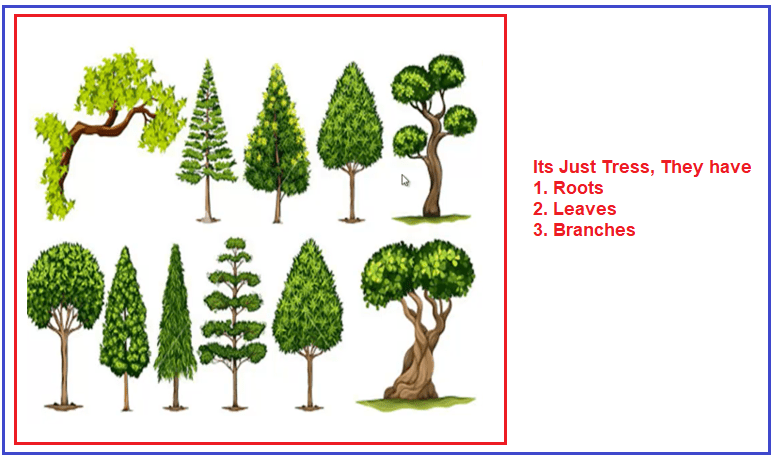In abstract thinking, we don’t have to go for each implementation and you know abstractly what you want to do. So, from a data structure point of view, instead of remembering all those 500 or 600 data structures, it would be great, if we group them into categories or abstract them into categories.

##### Example: Array

Let us think about the array. An array is one of the data structures. In array we have the following types:

1. One dimensional array
2. Two-dimensional array
3. Multi-Dimensional Array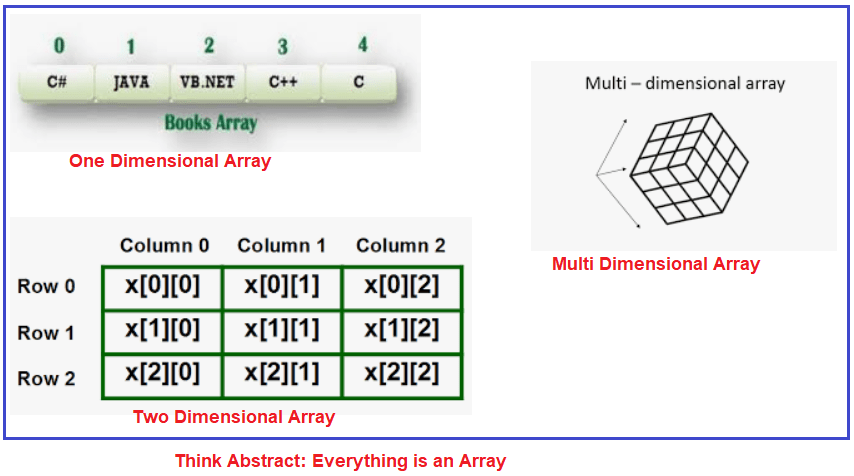Instead of remembering a one-dimension array, two-dimension array, multi-dimension array, think just abstract, it is an array. It can have values; it can have size. So, when you think about the abstract, then you don’t need to get into the implementation of each data structure.